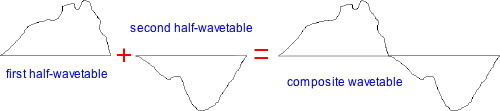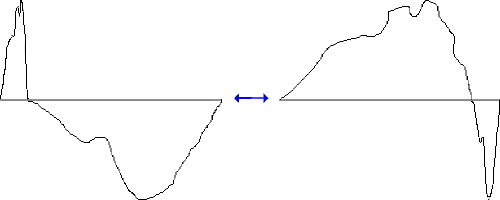RTcmix an open-source digital signal processing and sound synthesis language about · links · contact
HALFWAVE -- dual-wavetable synthesis instrument
in RTcmix/insts/bgg

quick syntax:
HALFWAVE(outsk, dur, PITCH, AMP, FIRSTHALF, SECONDHALF, WMIDPOINT[, PAN])

CAPITALIZED parameters are pfield-enabled for table or dynamic control (see the maketable or makeconnection scorefile commands). Parameters after the [bracket] are optional and default to 0 unless otherwise noted.

p0 = output start time (seconds)
p1 = duration (seconds)
p2 = pitch (Hz or oct.pc)
p3 = amplitude (absolute, for 16-bit soundfiles: 0-32768)
p4 = first half-wavetable reference
p5 = second half-wavetable reference
p6 = wavetable mid-crossover point [0.0-1.0]
p7 = pan (0-1 stereo; 0.5 is middle) [optional; default is 0]

p2 (pitch), p3 (amplitude), p6 (wavetable mid-crossover point) and p7 (pan)

p4 (first half-wavetable reference) and p8 (second half-wavetable reference),
if used, should be references to pfield table-handles.

HALFWAVE plays a waveform that is constructed in two halves. The 'join' point of the two half-waveforms can be dynamically modulated.

Usage Notes

Parameter p4 ("FIRSTHALF") should be a reference to a table containing half of the waveform to be constructed. Parameter p5 ("SECONDHALF") contains the second half. The two waveforms are joined like this:The composite waveform will be played at pitch p2 ("PITCH"). p2 can be either oct.pc or Hz (< 15.0 is the switch-over from Hz to oct.pc).

In addition, the 'join' point of the two waveforms (p6, "WMIDPOINT") can be dynamically modulated for timbral change throughout the note:When p6 is 0.0, the first half of the waveform (p4) will be completely "squished" to the left of the waveform; when p6 is 1.0 the p5 waveform will be "squished" to the right.

HALFWAVE can produce both mono and stereo output.

Sample Scores

very basic:
rtsetparams(44100, 1)

w1 = maketable("wave3", 1000, 0.5, 1, 0)
w2 = maketable("wave3", 1000, 0.5, 1, 180)

dex = maketable("line", 1000, 0,0, 1,1, 2,0.5)

HALFWAVE(0, 3.5, 8.00, 20000, w1, w2, dex)

rtsetparams(44100, 2)

amp = 3000
ampenv = maketable("line", 1000, 0,0, 1,1, 9,1, 10,0)

dur = 9.8

w1 = maketable("wave3", 1000, 0.5, 1, 0, 1, 0.2, 0, 3, 0.1, 0)
w2 = maketable("wave3", 1000, 0.5, 1, 180, 4, 0.5, 180, 7, 0.1, 180)

basepitch = 7.00
for (i = 0; i < 49; i += 1) {
dex = maketable("line", "nonorm", 1000, 0, random(), 1, random(), 2, random(), 3, random())

prange = 0.24
poff = maketable("line", "nonorm", 1000, 0, irand(0, prange),
0.5, irand(0, prange), 1.4, irand(0, prange),
2.5, irand(0, prange), 3, irand(0, prange), 5, irand(0, prange))

pan = maketable("line", "nonorm", 1000, 0, random(), 1, random(), 2, random(), 3, random())

HALFWAVE(0, dur, basepitch+poff, amp*ampenv, w1, w2, dex, pan)
}